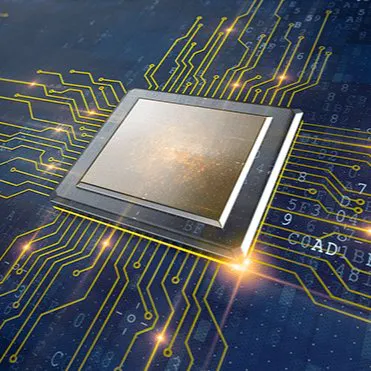# Smart and Sustainable Agriculture System

With climate change and water scarcity we will monitor farms Temperature, humidity, CO2 emissions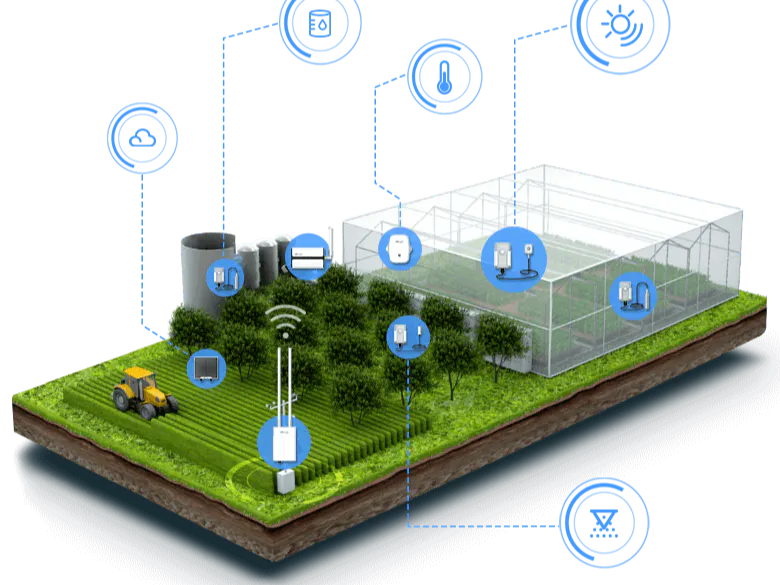## Things used in this project

### Hardware components

 NXP NAVQ+
×1
×1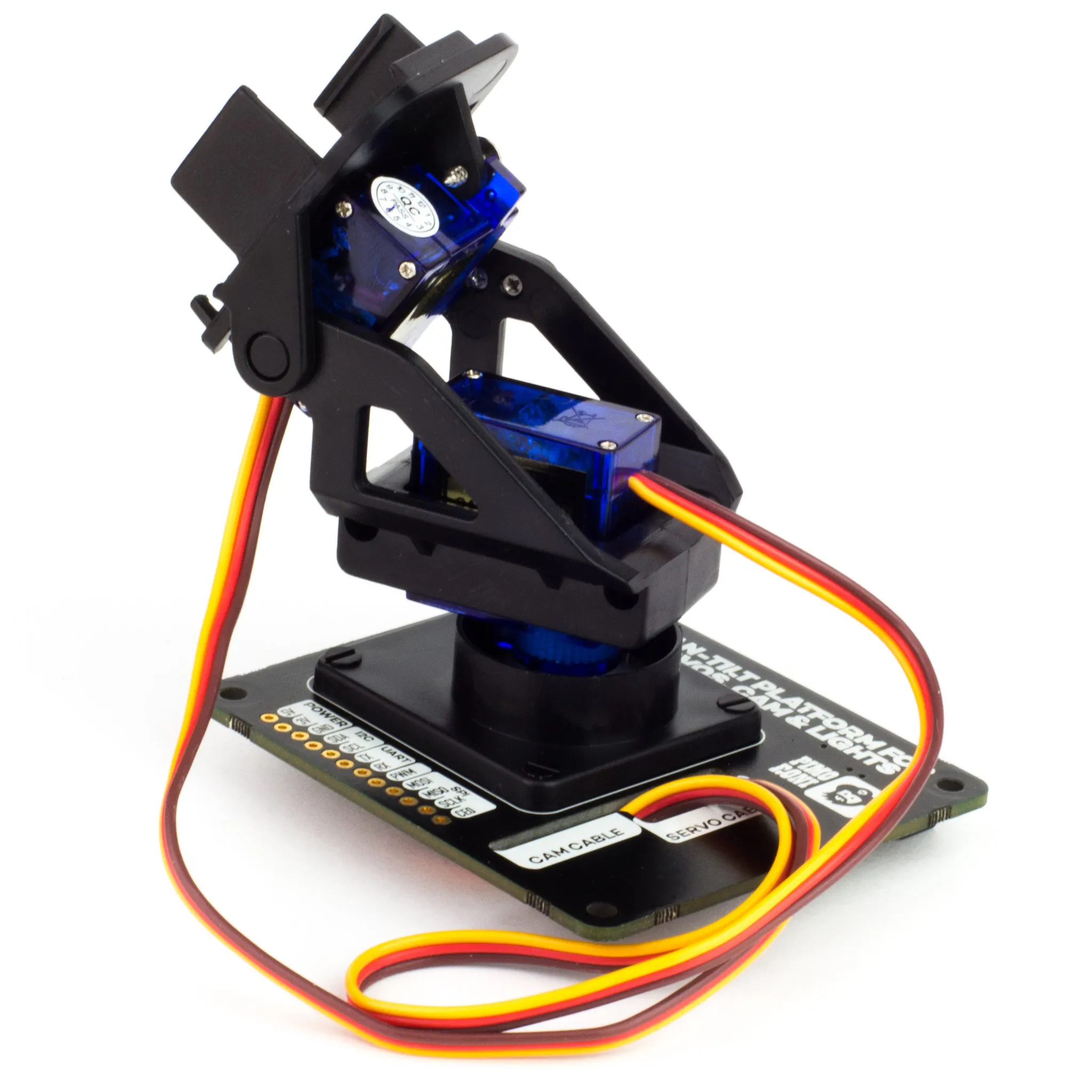Pimoroni Pan-Tilt HAT
×1
 Robot Smart Car 4WD
×1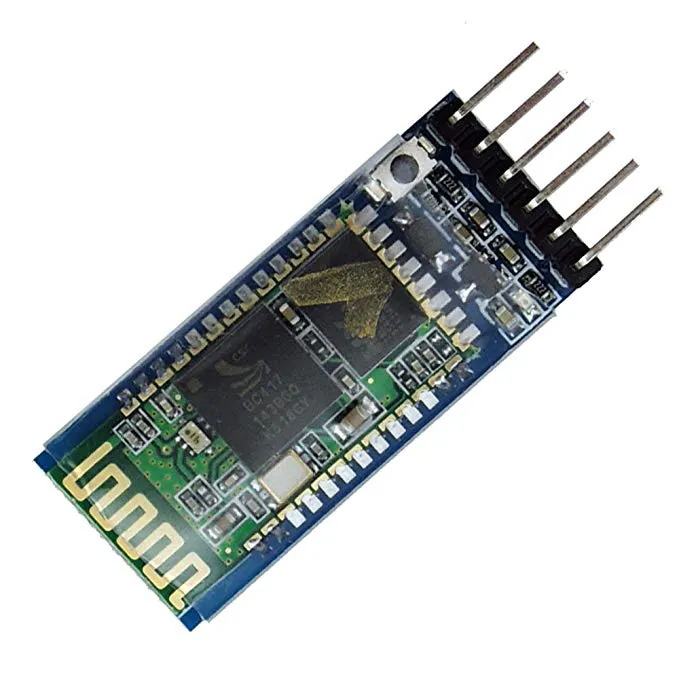HC-05 Bluetooth Module
×1Ultrasonic Sensor - HC-SR04 (Generic)
×1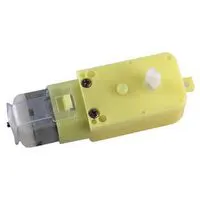DC Motor, 12 V
×1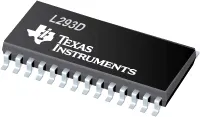Texas Instruments Dual H-Bridge motor drivers L293D
×1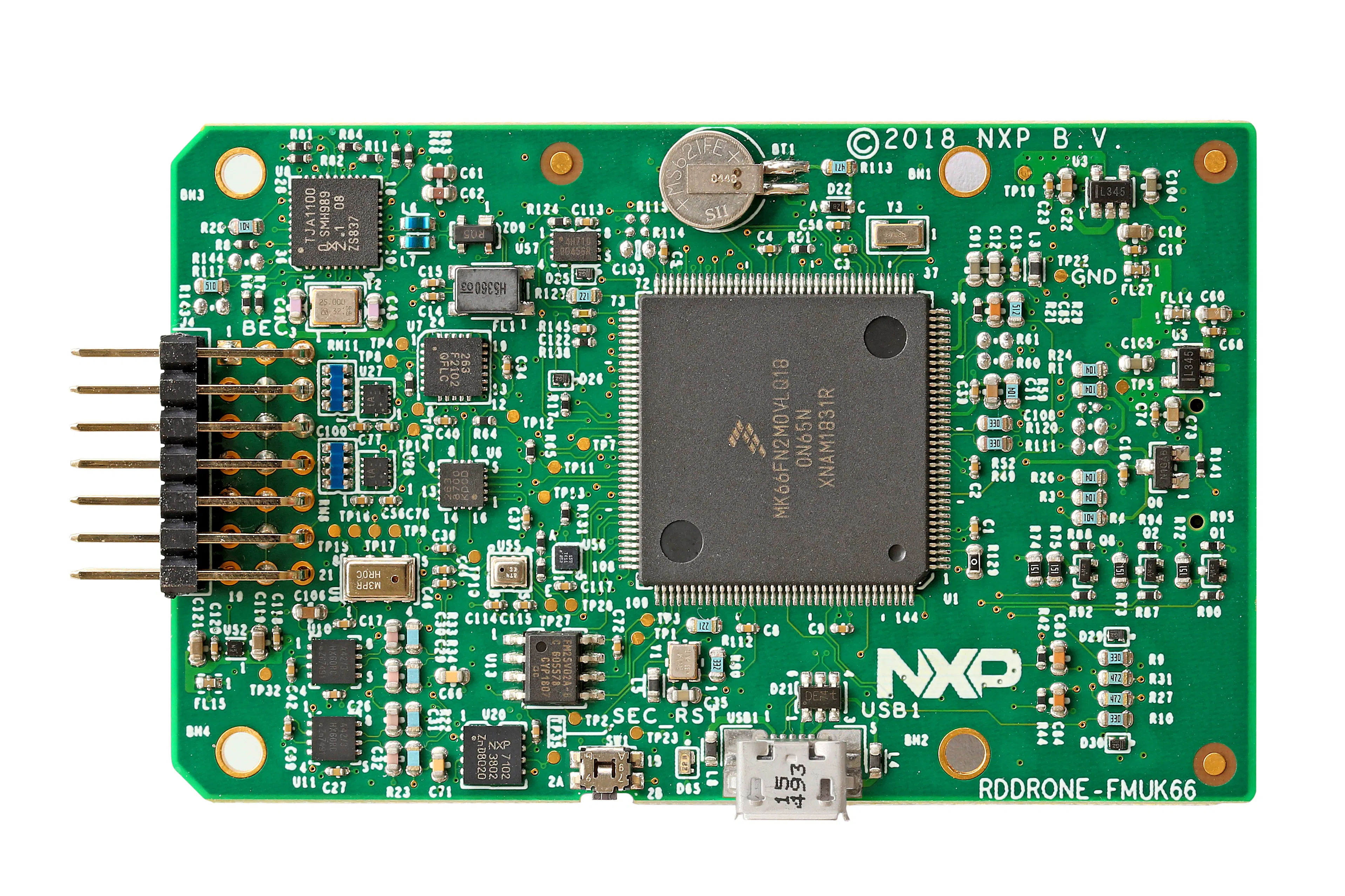NXP RDDRONE-FMUK66
×1

### Software apps and online servicesROS Robot Operating SystemSnappy Ubuntu CoreArduino IDE

### Hand tools and fabrication machines3D Printer (generic)

## Custom parts and enclosures

### NAVQ+ ENCLUSER

references
https://iroboteducation.github.io/create3_docs/hw/print_compute/#navqplus-by-nxp

### NAVQ+ ENCLUSER TOP

reference
https://iroboteducation.github.io/create3_docs/hw/print_compute/#navqplus-by-nxp

### NAVQ+ ENCLUSER BOTTOM

reference
https://iroboteducation.github.io/create3_docs/hw/print_compute/#navqplus-by-nxp

## Schematics

### ROBOT CONTROL ARDUINO NANO

ROBOT CONTOL TO CONTROL THE 4 WHEEL ROBOT THROUGH I2C FROM NAVQ+ OR BLUETOOTH FROM SMART PHONE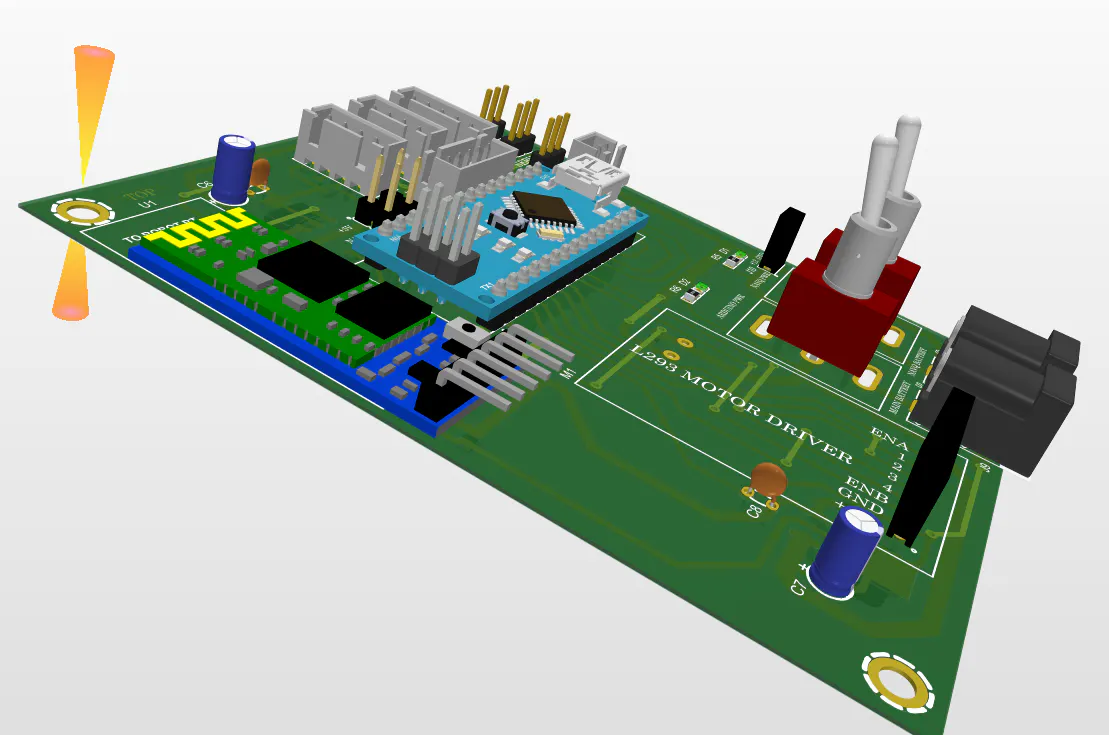### arduino based robot controller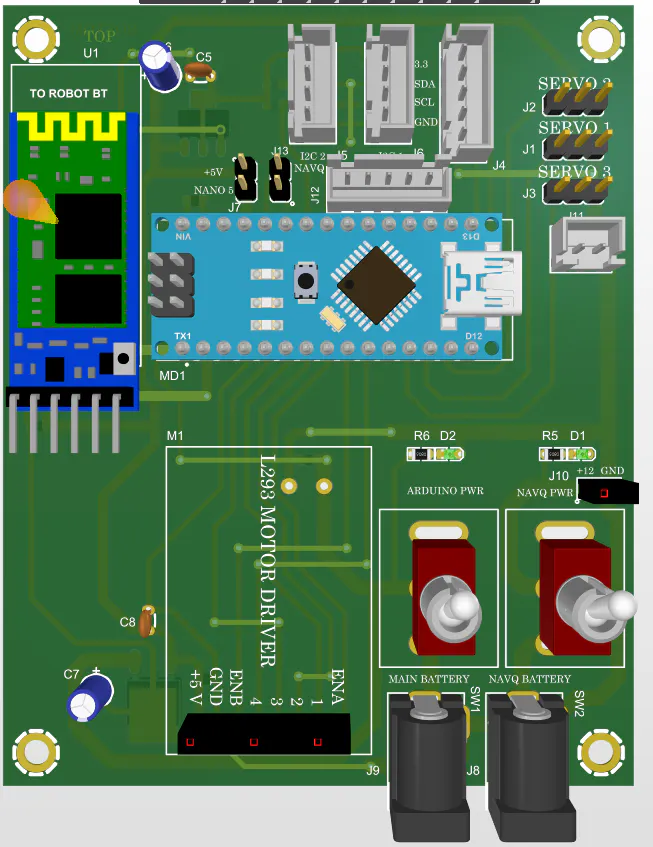### arduino based robot controller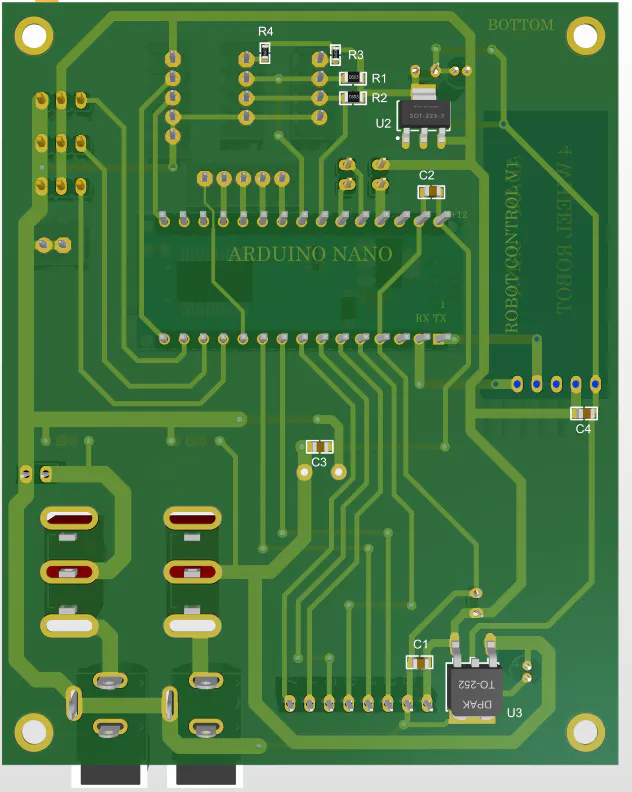## Code

### arduino based robot controller

C/C++
```#include <AFMotor.h>
#include <Wire.h>
#include <Servo.h>
#include <SoftwareSerial.h>
#define trigpin 12
#define echopin 13
#define ENA 5
#define ENB 6
#define IN1 3
#define IN2 4
#define IN3 2
#define IN4 7
Servo pan; //pan tilt azimus
Servo tilt; // pan tilt elevation
int distance_L, distance_F, distance_R;
long distance;
long data(void);
int set = 20;
int speed =255;
int x = 0;
//float cm; //Distance variable
//float temp; //
SoftwareSerial mySerial(8, 17); // RX, TX
void setup() {
// Start the I2C Bus as Slave on address 9
Wire.begin(50);
// Attach a function to trigger when something is received.
Serial.begin(9600);
// set the data rate for the SoftwareSerial port
mySerial.begin(9600);
mySerial.println("Hello Hover Gamers");
pinMode(trigpin, OUTPUT);
pinMode(echopin, INPUT);

pan.attach(10);
tilt.attach(11);

pan.write(70);
tilt.write(50);
pinMode(IN1, OUTPUT);
pinMode(IN2, OUTPUT);
pinMode(IN3, OUTPUT);
pinMode(IN4, OUTPUT);

pinMode(ENA, OUTPUT);
pinMode(ENB, OUTPUT);
}
Serial.println(x);
}
void loop() {
// STOP();
///////////////////////////////////////////////////////////////////////////
if (x == 'F') {
Serial.println("Forward");
forward(); // turn it on going forward
delay(500);
x =0;
}
else if (x == 'B') {
Serial.println("REVERSE");
back();                    // turn it on going forward
delay(500);
x =0;
}
else if (x == 'L') {
Serial.println("LEFT");
Left();                    // turn it on going forward
delay(500);
x =0;
}
else if (x == 'R') {
Serial.println("RIGHT");
Right();                    // turn it on going forward
delay(500);
x =0;
}
else if (x == 'S') {
Serial.println("STOP");
STOP();                    // turn it on going forward
delay(500);
x =0;
}
else if (x == 'W') {
Serial.println("nospeed");
speed =0;                    // turn it on going forward
//delay(500);
x =0;
}
else if (x == 'X') {
Serial.println("LOW SPEED");
speed =170;                    // turn it on going forward
//delay(500);
x =0;
}
else if (x == 'Y') {
Serial.println("REGULAR SPEED");
speed =200;                    // turn it on going forward
//delay(500);
x =0;
}
else if (x == 'Z') {
Serial.println("HIGH SPEED");
speed =255;                    // turn it on going forward
//delay(500);
x =0;
}
///////////////////////PAN CONTROL////////////////////////////////
else if (x == 'a') {
Serial.println("center");
pan.write(70);                    // turn it on going forward
//delay(500);
x =0;
}
else if (x == 'b') {
Serial.println("leftpan");
pan.write(170);                    // turn it on going forward
//delay(500);
x =0;
}
else if (x == 'c') {
Serial.println("rightpan");
pan.write(7);                    // turn it on going forward
//delay(500);
x =0;
}
else if (x == 'd') {
Serial.println("left c");
pan.write(120);                    // turn it on going forward
//delay(500);
x =0;
}
else if (x == 'e') {
Serial.println("right c");
pan.write(35);                    // turn it on going forward
//delay(500);
x =0;
}

///////////////////////Tilt CONTROL////////////////////////////////
else if (x == 'g') {
Serial.println("center tilt");
tilt.write(70);                    // turn it on going forward
//delay(500);
x =0;
}
else if (x == 'h') {
Serial.println("downtilt");
tilt.write(110);                    // turn it on going forward
//delay(500);
x =0;
}
else if (x == 'i') {
Serial.println("up tilt");
tilt.write(7);                    // turn it on going forward
//delay(500);
x =0;
}
else if (x == 'j') {
Serial.println("down center");
tilt.write(120);                    // turn it on going forward
//delay(500);
x =0;
}
else if (x == 'k') {
Serial.println("up center");
tilt.write(35);                    // turn it on going forward
//delay(500);
x =0;
}
else{
STOP();
}
////////////////////////////////////////////////////////////////////////////
// this is the main function routine for autonomous car drive
/*
distance_F = data();
Serial.print("S=");
Serial.println(distance_F);
if (distance_F > set)
{
Serial.println("Forward");
forward();                    // turn it on going forward
}
else
{
hc_sr4();
}

if (distance_F < 10)
{
STOP();
}
*/
}

long data(){
digitalWrite(trigpin, LOW);
delayMicroseconds(2);
digitalWrite(trigpin, HIGH);
delayMicroseconds(10);
distance = pulseIn (echopin, HIGH);
return distance / 29 / 2;
}

//digitalWrite(Trig, LOW);
//delayMicroseconds(2);
// digitalWrite(Trig, HIGH);
// delayMicroseconds(10);
// digitalWrite(Trig, LOW);

//  temp = float(pulseIn(Echo, HIGH));
//  cm = (temp * 17 ) / 1000;
//  if (cm < 25 && cm > 10)
//  {
//    back();
//    delay(500);
//    Left();
//    delay(200);
//  }
//  if (cm >= 25)
//  {
//    forward();
//    delay(100);
//  }
//
//
//  if (cm < 10)
//  {
//    STOP();
//  }
//  Serial.print("Echo =");
//  Serial.print(temp);
//  Serial.print(" | | Distance = ");
//  Serial.print(cm);
//  Serial.println("cm");
//  delay(100);
//}

void compareDistance(){
if (distance_L > distance_R){
back();
delay(200);
Left();   // turn it on going left
delay(350);
}
else if (distance_R > distance_L){
back();
delay(200);
Right();  // the other right
delay(350);
}
else{
back();  // the other way

delay(300);
Left();   // turn it on going left

delay(500);
}
}

void hc_sr4(){
Serial.println("Stop");
STOP();         // stopped

pan.write(10);
delay(300);
distance_R = data();
delay(100);
pan.write(170);
delay(500);
distance_L = data();
delay(100);
pan.write(70);
delay(300);
compareDistance();
}
void forward() {
analogWrite(ENA, speed);
analogWrite(ENB, speed);

digitalWrite(IN1, HIGH);
digitalWrite(IN2, LOW);
digitalWrite(IN3, LOW);
digitalWrite(IN4, HIGH);
Serial.println("Forward");
}

void back() {
analogWrite(ENA, speed);
analogWrite(ENB, speed);
digitalWrite(IN1, LOW);
digitalWrite(IN2, HIGH);
digitalWrite(IN3, HIGH);
digitalWrite(IN4, LOW);
Serial.println("Back");
}

void Left() {
analogWrite(ENA, speed);
analogWrite(ENB, speed);
digitalWrite(IN1, LOW);
digitalWrite(IN2, HIGH);
digitalWrite(IN3, LOW);
digitalWrite(IN4, HIGH);
Serial.println("Left");
}

void Right() {
analogWrite(ENA, speed);
analogWrite(ENB, speed);
digitalWrite(IN1, HIGH);
digitalWrite(IN2, LOW);
digitalWrite(IN3, HIGH);
digitalWrite(IN4, LOW);
Serial.println("Right");
}

void STOP() {
digitalWrite(ENA, LOW);
digitalWrite(ENB, LOW);
digitalWrite(IN1, LOW);
digitalWrite(IN2, LOW);
digitalWrite(IN3, LOW);
digitalWrite(IN4, LOW);
Serial.println("STOP");
}
```